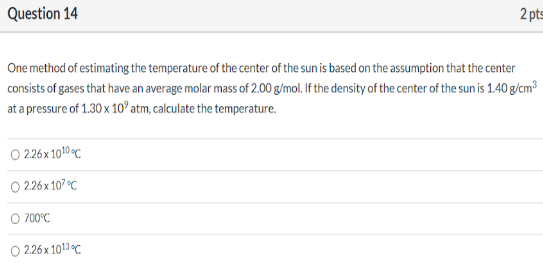# One method of estimating the temperature of the center of the sun is based on the assumption that the center consists of gasses that have an average molar mass of 2.00 g/mol. If the density of the center of the sun is 1.40 g/cm^3 at a pressure of 1.30x10^9 atm, calculate the temperature. a) 2.26x10^10 degrees C b) 2.26x10^7 degrees C c) 700 degrees C d) 2.26x10^13 degrees C